Processing ......FreeComputerBooks.com Links to Free Computer, Mathematics, Technical Books all over the World

First Course in the Theory of Equations
Top Free Computer Networking Books 🌠 - 100% Free or Open Source!
• Title: First Course in the Theory of Equations
• Author(s) Leonard Eugene Dickson
• Publisher: The Project Gutenberg
• Permission: This eBook is for the use of anyone anywhere at no cost and with almost no restrictions whatsoever. You may copy it, give it away or re-use it under the terms of the Project Gutenberg License included with this eBook or online at www.gutenberg.org
• Paperback: N/A
• eBook: PDF, ePub, Kindle, TeX, etc.
• Language: English
• ISBN-10: N/A
• ISBN-13: N/A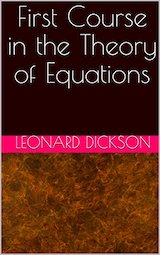Book Description

The theory of equations is not only a necessity in the subsequent mathematical courses and their applications, but furnishes an illuminating sequel to geometry, algebra and analytic geometry. Moreover, it develops anew and in greater detail various fundamental ideas of calculus for the simple, but important, case of polynomials. The theory of equations therefore affords a useful supplement to differential calculus whether taken subsequently or simultaneously.

Reviews, Ratings, and Recommendations: Related Book Categories: Read and Download Links:Similar Books:
•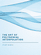The Art of Polynomial Interpolation (Stuart Murphy)

Exploring the techniques of interpolating data allows us to view the development and birth of a polynomial. This book is focused on laying a foundation for understanding and applying several common forms of Polynomial Interpolation.

•Tea Time Numerical Analysis: Experiences in Mathematics

This textbook was born of a desire to contribute a viable, free, introductory Numerical Analysis textbook. The ultimate goal of this book is to be a complete, one-semester, single-pdf, downloadable textbook designed for mathematics classes.

•Numerical Algorithms: Computer Vision, Machine Learning, etc.

This book presents a new approach to numerical analysis for modern computer scientists, covers a wide range of topics - from numerical linear algebra to optimization and differential equations - focusing on real-world motivation and unifying themes.

•Numerical Methods and MATLAB Programming for Engineers

It covers the fundamentals while emphasizing the most essential numerical methods. Readers will enhance their programming skills using MATLAB to implement algorithms, discover how to use MATLAB to solve problems in science and engineering.

•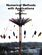Numerical Methods with Applications, 2nd Edition (Autar K Kaw)

This book entitled Numerical Methods with Applications is written primarily for engineering undergraduates taking a course in Numerical Methods. It offers a unique treatise to numerical methods which is based on a holistic approach and short chapters.

•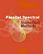Parallel Spectral Numerical Methods (Gong Chen, et al)

This book teaches the principals of Fourier spectral methods, their utility in solving partial differential equation and how to implement them in code. The programs will use Matlab and Fortran. A Python implementation of some of the Matlab programs is also provided.

•Measure, Integration and Real Analysis (Sheldon Axler)

This textbook welcomes students into the fundamental theory of measure, integration, and real analysis. Focusing on an accessible approach, it lays the foundations for further study by promoting a deep understanding of key results.

•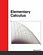Elementary Calculus (Michael Corral)

This textbook covers calculus of a single variable, is designed for students who have completed courses in high-school algebra, geometry, and trigonometry. Though designed for college students, it could also be used in high schools.

•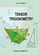Tensor Trigonometry (A.S. Ninul)

The tensor trigonometry is development of the flat scalar trigonometry from Leonard Euler classic forms into general multi-dimensional tensor forms with vector and scalar orthoprojections and with step by step increasing complexity and opportunities.

•Computational Linear Algebra and N-dimensional Geometry

This undergraduate textbook on Linear Algebra and n-Dimensional Geometry, in a self-teaching style, is invaluable for sophomore level undergraduates in mathematics, engineering, business, and the sciences.

Book Categories
 :All CategoriesTop Free BooksRecent BooksMiscellaneous BooksComputer EngineeringComputer LanguagesComputer ScienceData Science/DatabasesJava and Java EE (J2EE)Linux and UnixMathematicsMicrosoft and .NETMobile ComputingNetworking and CommunicationsSoftware EngineeringSpecial TopicsWeb Programming
Other Categories